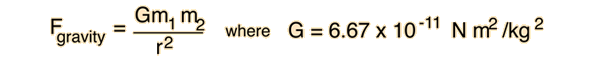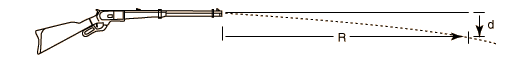Index HyperPhysics***** Mechanics Go Back

# Gravity

Gravity is the weakest of the four fundamental forces, yet it is the dominant force in the universe for shaping the large scale structure of galaxies, stars, etc. The gravitational force between two masses m1 and m2 is given by the relationship:This is often called the "universal law of gravitation" and G the universal gravitation constant. It is an example of an inverse square law force. The force is always attractive and acts along the line joining the centers of mass of the two masses. The forces on the two masses are equal in size but opposite in direction, obeying Newton' s third law. Viewed as an exchange force, the massless exchange particle is called the graviton.

The gravity force has the same form as Coulomb's law for the forces between electric charges, i.e., it is an inverse square law force which depends upon the product of the two interacting sources. This led Einstein to start with the electromagnetic force and gravity as the first attempt to demonstrate the unification of the fundamental forces. It turns out that this was the wrong place to start, and that gravity will be the last of the forces to unify with the other three forces. Electroweak unification (unification of the electromagnetic and weak forces) was demonstrated in 1983, a result which could not be anticipated in the time of Einstein's search. It now appears that the common form of the gravity and electromagnetic forces arises from the fact that each of them involves an exchange particle of zero mass, not because of an inherent symmetry which would make them easy to unify.

Index

Gravity Concepts
HyperPhysics***** Mechanics Go Back

# Examples of Trajectories

## A dropped bullet will hit the ground before one which is fired from a gun.

As shown in the illustration of a horizo= ntal launch, gravity acts the same way on both bullets, giving them the same downward acceleration and making them strike the ground at the same time if the bullet is fired horizontally over level ground.

## Bullets fired from high-powered rifles drop only a few inches in hundreds of yards.

Fired at twice the speed of sound, a bullet will drop over 3 inches in 100 yards, and at 300 yards downrange will have dropped about 30 inches. Plug in numbers into the bullet drop calculation to see for yourself. Ammunition manufacturers contribute to this misconception by stating the drop of their projectiles as just the extra drop caused by frictional drag compared to an ideal frictionless projectile.
Index

Trajectory concepts

HyperPhysics***** Mechanics Go Back

# Drop of a BulletIf air friction is neglected, then the drop of a bullet fired horizontally can be treated as an ordinary horizontal trajectory. The air friction is significant, so this is an idealization.

If the muzzle velocity is
v m/s ft/s mi/hr km/hr

and the distance downrange is
R m ft yards,

Then the amount of drop of the bullet below the horizontal would be
d m cm inches

If the gun is fired on level ground at a height of m ft, then the bullet will hit the ground in seconds, having traveled a distance of meters feet.

To hold the drop to cm inches at the downrange distance R above would require a muzzle velocity of m/s ft/s.

Index

Trajectory concepts

HyperPhysics***** Mechanics Go Back